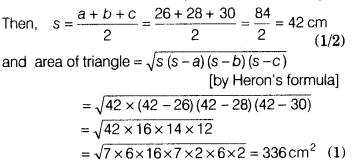# If the sides of the triangle are 26 cm, 28 cm and 30 cm and the parallelogram

A triangle and a parallelogram have the same base and the same area. If the sides of the triangle are 26 cm, 28 cm and 30 cm and the parallelogram stands on the base 28 cm, find the height of the parallelogram.

Let the sides of triangle be a = 26 cm, b = 28 cm and c = 30 cm. Let s be the semi-perimeter of the triangle.Let h be the height of the parallelogram.
Then, area of parallelogram = base x height = 28xh
∵ Area of parallelogram = Area of triangle [given]
∴ 28xh = 366 ⇒ h = 336/28⇒ h=12cm
Hence, the height of the parallelogram is 12 cm.